### Home > MC2 > Chapter 10 > Lesson 10.1.1 > Problem10-11

10-11.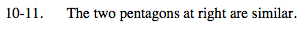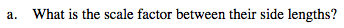Compare the ratio of two of the given corresponding sidesfrom the old to the new shape. What do you get?

$\frac{6}{9} = \frac{4}{6} = \frac{2}{3}$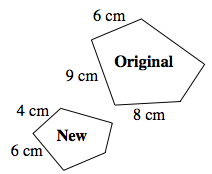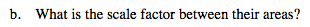$\text{The area ratio is } \frac{\text{new area}}{\text{original area}} = ( \text{scale factor} )^2$

$\text{If the scale factor is }\frac{2}{3}, \text{ what would the area ratio be?}$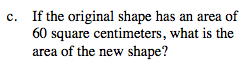Use the area ratio you found in part (b) to determine the area of the new shape.

Multiply original area by the area ratio to get new area.

26.67 cm2